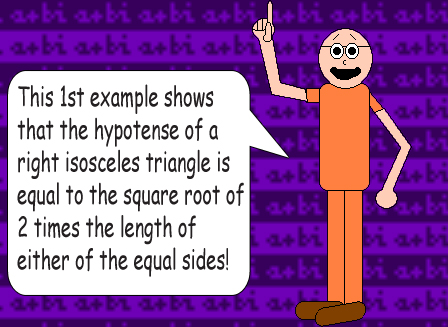# Pythagorean Power: Exponential Excellence

## This math trick is like an extended version of the Pythagorean Theorem!## The examples below show why!

(x^2 + x^2)^½ = (2x^2)^½ = x * (2)^½ or x * 1.4142...

(That irrational number is the square root of 2)

The caret (^) is the symbol of exponentiation. Raising a number to the power of ½ gives you the square root.## What number is x equal to in all these examples? Any number you want it to be!

(x^2 + x^2 + x^2)^½ = (3x^2)^½ = x * (3)^½ or x * 1.7320...

(That irrational number is the square root of 3)

(x^2 + x^2 + x^2 + x^2)^½ = (4x^2)^½ = x * (4)^½ or 2x

(4 has another integer for a square root, which is 2)

(x^2 + x^2 + x^2 + x^2 + x^2)^½ = (5x^2)^½ = x * (5)^½ or x * 2.2360...

(That irrational number is the square root of 5)

### If y has a rational square root, then x will be multiplied by a rational number!

Remember that multiplication is actually glorified addition. In the function on top of this page, the variable y tells you how many times you should add x (or rather its square) to itself. In other words, if y = 7, then x is multiplied by 7 so you get x + x + x + x + x + x + x = 7x. Exponentiation is glorified multiplication. (x * x * x = x^3) In algebra, exponentiation is usually done before multiplication, which is usually done before addition; higher calculation levels are done before lower ones. However, parentheses tell us to do the math between them first before doing any math on the outside!

Addition & subtraction are on the same level; the lowest level of calculation. The next higher level is multiplication & division; then exponentiation, radicals & logarithms, which is the highest. This is called the "Please Excuse My Dear Aunt Sally" Rule.